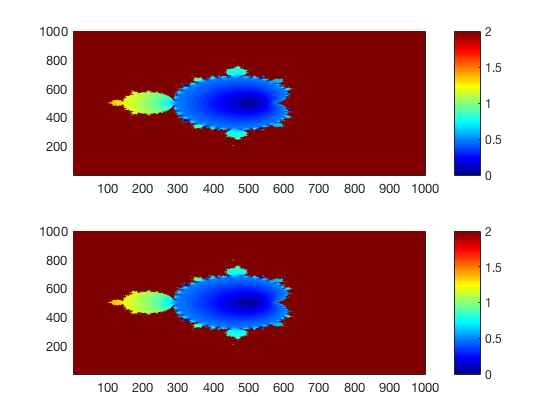# Session 2: Speed-up your code by vectorization¶

Date: 09/18/2017, Monday

## For loops¶

You already know how to create a Mandelbrot set by writting tons of “for” loops. If not, see Lecture 5’s note.

In :

%%file mande_by_loops.m
function Z_final = mande_by_loops(C, T)

[nx,ny] = size(C);
Z_final = zeros(nx,ny); % to hold last value of z, at all possible points.

for ix = 1:nx
for iy = 1:ny

% get the value of c at current point.
% note that MATLAB is case-sensitive
c = C(ix,iy);
z = 0; % initial value, doesn't matter too much
for t=2:T
z = z^2+c;
end
Z_final(ix,iy) = z; % save the last value of z

end
end

end

Created file '/Users/zhuangjw/Research/Computing/personal_web/AM111/docs/mande_by_loops.m'.


## Vectorization¶

The above function has 3 “for” loops, but 2 of them are not necessary, because you can operate on the entire array.

In :

%%file mande_by_vec.m
function Z = mande_by_vec(C, T)
% vectorized over C and Z

[nx,ny] = size(C);
Z = zeros(nx,ny);
for t=2:T
Z = Z.^2+C;
end

end

Created file '/Users/zhuangjw/Research/Computing/personal_web/AM111/docs/mande_by_vec.m'.


## Performance comparision¶

Compared to the for-loop version, this vectorized version is much shorter, and 20x faster!

In :

% initialization
nx = 1000;
xm = 1.75;
x = linspace(-xm, xm, nx);
y = linspace(-xm, xm, nx);
[Cr, Ci] = meshgrid(x,y);
C = Cr + i*Ci;

T = 50;

% use loops
tic
Z_loop = mande_by_loops(C,T);
toc

% use vectorization
tic
Z_vec = mande_by_vec(C,T);
toc

% check if results are equal
isequal(Z_vec,Z_loop)

% plot two results
subplot(211);pcolor(abs(Z_loop));

subplot(212);pcolor(abs(Z_vec));

Elapsed time is 4.883975 seconds.
Elapsed time is 0.255324 seconds.

ans =

logical

1MATLAB’s loop is notoriously slow because it keeps checking the variable types at every iteration. A rule of thumb is shorter code is often faster, in terms of achieving the same functionality. ( Only for high-level languages like MATLAB and Python. Loop is fast in low-level languages.)